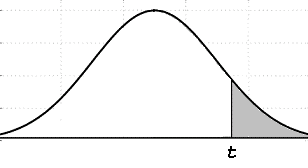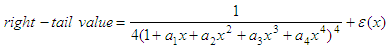# Student's t distribution

 This algorithm (the Matlab code developed below) calculates right-tail values for points on a t-distribution curve. You must provide the value of t and the degrees of freedom. The t-distribution is a continuous distribution that arises when estimating the mean of a normally distributed population in situations where the sample size is small.Student's t-distribution curve

The shaded area represents the right-tail value for t.

The right-tail value is approximated using the following formula:where

a1 = 0.196854
a2 = 0.115194
a3 = 0.000344
a4 = 0.019527d = degrees of freedomThe Matlab program is:

% Clears screen and deletes all the variables in the workspace
clc; clear;

% Asks the user for input
t = input('T-value: ');
d = input(
'Degrees of freedom: ');

x = 1;
y = 1;
t = t^2;

% Computes using inverse for small T-values
if t < 1
s = d;
r = y;
z = 1/t;

else
s = y;
r = d;
z = t;

end

j = 2/(9*s);
k = 2/(9*r);

% Computes using approximation formulas
l = abs((1 - k)*z^(1/3) - 1 + j)/sqrt(k*z^(2/3) + j);

if r < 4
l = l*(1 + 0.08*l^4/r^3);

end

a1 = 0.196854;
a2 = 0.115194;
a3 = 0.000344;
a4 = 0.019527;
x = 0.25/(1 + l*(a1 + l*(a2 + l*(a3 + l*a4))))^4;
x = floor(x*10000 + 0.5)/10000;

% Adjusts if inverse was calculated
if t < 1
x = 1 - x;

end

% Displays result
str = ['Rigth tail value: ' num2str(x)];
disp(str)

Example 1:

What is the right-tail value when the t-value is 2.921 and there are 16 degrees of freedom?

We run the code above and enter data:

T-value: 2.921
Degrees of freedom: 16

Rigth tail value: 0.0049

Example 2:

T-value is 11.178, with 5 degrees of freedom. What’s the tail value?

Running the program and entering appropriate data, we get:

T-value: 11.178
Degrees of freedom: 5
Rigth tail value: 0.0002

From 't distribution' to home

From 't distribution' to 'Probability and Statistics' Menu